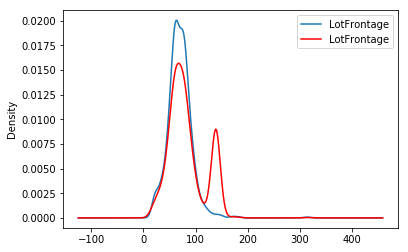# EndTailImputer#

The `EndTailImputer()` replaces missing data with a value at the end of the distribution. The value can be determined using the mean plus or minus a number of times the standard deviation, or using the inter-quartile range proximity rule. The value can also be determined as a factor of the maximum value.

You decide whether the missing data should be placed at the right or left tail of the variable distribution.

In a sense, the `EndTailImputer()` “automates” the work of the `ArbitraryNumberImputer()` because it will find automatically “arbitrary values” far out at the end of the variable distributions.

`EndTailImputer()` works only with numerical variables. You can impute only a subset of the variables in the data by passing the variable names in a list. Alternatively, the imputer will automatically select all numerical variables in the train set.

Below a code example using the House Prices Dataset (more details about the dataset here).

First, let’s load the data and separate it into train and test:

```import numpy as np
import pandas as pd
import matplotlib.pyplot as plt
from sklearn.model_selection import train_test_split

from feature_engine.imputation import EndTailImputer

# Separate into train and test sets
X_train, X_test, y_train, y_test = train_test_split(
data.drop(['Id', 'SalePrice'], axis=1),
data['SalePrice'],
test_size=0.3,
random_state=0,
)
```

Now we set up the `EndTailImputer()` to impute in this case only 2 variables from the dataset. We instruct the imputer to find the imputation values using the mean plus 3 times the standard deviation as follows:

```# set up the imputer
tail_imputer = EndTailImputer(imputation_method='gaussian',
tail='right',
fold=3,
variables=['LotFrontage', 'MasVnrArea'])
# fit the imputer
tail_imputer.fit(X_train)
```

With fit, the `EndTailImputer()` learned the imputation values for the indicated variables and stored it in one of its attributes. We can now go ahead and impute both the train and the test sets.

```# transform the data
train_t= tail_imputer.transform(X_train)
test_t= tail_imputer.transform(X_test)
```

Note that after the imputation, if the percentage of missing values is relatively big, the variable distribution will differ from the original one (in red the imputed variable):

```fig = plt.figure()In the following Jupyter notebook you will find more details on the functionality of the `CategoricalImputer()`, including how to select numerical variables automatically, how to impute with the most frequent category, and how to impute with a used defined string.